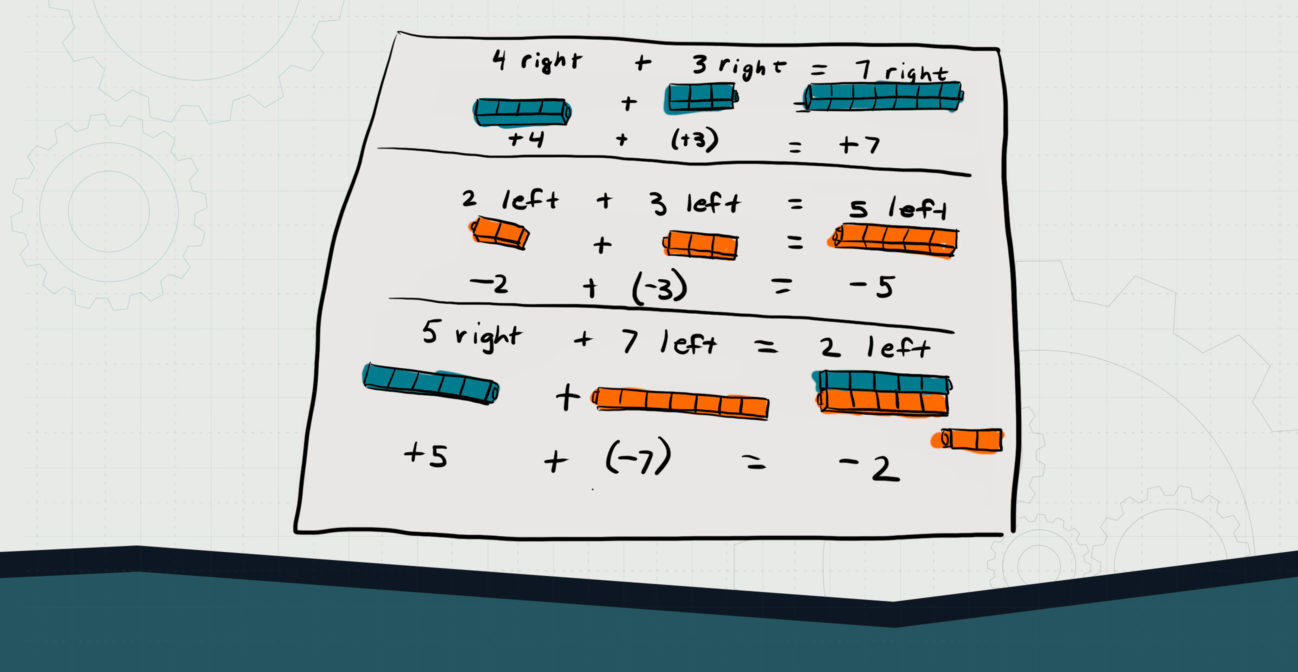# Vector-y is Ours

## Use bricks to investigate vector addition.Objective:
Child will be able to use SOHO Bricks as manipulatives to add and subtract vectors.

Essential Question:
How might we add and subtract vectors of varying directions?

Special Materials:
None

Bricks Required:

1×1 bricks of different colors

Project Structure

Engage/Explain:

1. Provide child with 1×1 SOHO bricks in various colors.
2. Ask child to construct several vectors of various magnitude and direction (“4 bricks to the right” “7 bricks to the left”).
3. Ask child to solve several vector addition problems using their bricks to model the vector additions (it is a good idea to start with same-direction vectors and then proceed to opposite-direction vectors).
4. Have child write brief explanations of how their vector addition prob

Explore:

1. Provide more rigorous problems to child by giving them horizontal and vertical vector problems that can be modeled on graph paper.
2. Ask child to develop a method for determining how to add these vectors together.
3. Work with child on developing the parallelogram rule and using tip-to-tail methods of lining up vectors.
4. Child should practice solving problems visually, as well as numerically.

Explain/Elaborate

1. Provide child with problem sets to practice adding and subtracting a series of vector problems that can be solved numerically and with graphing.
2. Child should also demonstrate graphically how vectors a+b = b+a.